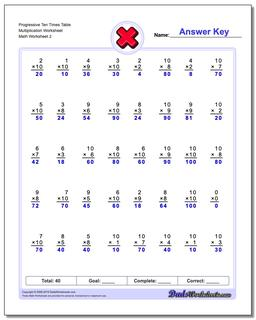Math Worksheets

### Worksheet News

PLEASE GO BACK AND USE THE BIG BLUE 'PRINT' BUTTON ON THE PAGE TO PRINT THE WORKSHEET CORRECTLY!Sorry for the trouble! The browser won't print the embedded worksheet PDF directly using the normal 'Print' command in the file menu, so you need to click the big 'Print' button to send just the worksheet and not the surrounding page to the printer.

# Math Worksheets: Multiplication: Multiplication: Progressive Ten Times Table Multiplication Worksheet (Second Worksheet)## Progressive Ten Times Table Multiplication Worksheet (Second Worksheet)

Property Value
DescriptionProgressive Ten Times Table Multiplication Worksheet: These are progressive multiplication worksheets for incrementally building multiplication facts, with facts grouped by a common multiplicand. Start with the ones time table, and then progress through the two times table, three times table, etcetera, all the way through the nine times table. 40 problem timed test format. (Second Worksheet)
Resource TypeWorksheet